# Class 11 Maths NCERT Solutions for Chapter 4 Principle of Mathematical Induction Exercise 4.1### Principle of Mathematical Induction Exercise Solutions

1. Prove the following by using the principle of mathematical induction for all n ∈ N:
1 + 3 + 1 + 3 + 32 + ….. + 3n-1 = (3n – 1)/2

Solution

Let the given statement be P(n), i.e.,
P(n): 1 + 3 + 32 + …. + 3n-1 = (3n – 1)/2
For n = 1 we have
P(1): 1 = (31 – 1)/2 = (3-1)/2 = 2/2 = 1, which is true.
Let P(k) be true for some positive integer k, i.e.,
1 + 3 + 32 + …. + 3k-1 = (3k – 1)/2  ...(i)
We shall now prove that P(k + 1) is true.
consider
1 + 3 + 32 + ….+ 3k-1 + (3(k+1)-1
= (1 + 3 + 32 + .... + 3k - 1) + 3k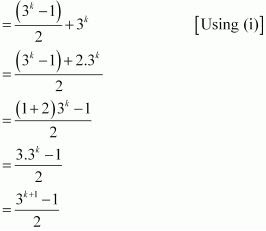Thus, P(k + 1) is true whenever P(k) is true.
Hence, by the principle of mathematical induction, statement P(n) is true for all natural numbers i.e., n.

2. Prove the following by using the principle of mathematical induction for all n ∈ N: 13 + 23 + 33+ ….+ n3 = [n(n+1)/2]2
Solution
Let the given statement be P(n) , i.e.,
P(n) : 13 + 23 + 33 + …. + n3 = [n(n+1)/2]2
For n = 1, we have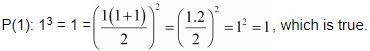Let P(k) be true for some positive integer k, i.e.,
13 + 23 + 33 + ….+ k3 = [k(k+1)/2]2  ...(i)
We shall now prove that P(k + 1) is true.
Consider
13 + 23 + 33 + ....+ k3 + (k + 1)3
= (13 + 23 + 33 + .... + k3 ) + (k + 1)3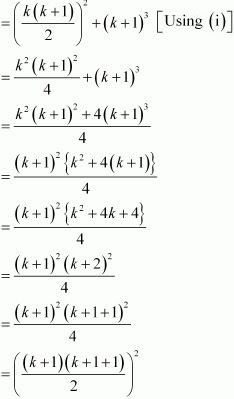Thus, P(k + 1) is true whenever P(k) is true.
Hence, by the principle of mathematical induction, statement P(n) is true for all natural number i.e., n.

3. Prove that following by using the principle of mathematical induction for all n ∈ N :  1 + 1/(1 + 2) + 1/(1 + 2 + 3) + .... + 1/(1 + 2 + 3 + ...n) = 2n/(n + 1)
Solution
Let the given statement be P(n), i.e.,
P(n):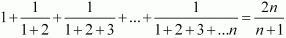For n = 1, we have
P(1) : 1 = 2.1/(1 + 1) = 2/2 = 1 which is true.
Let P(k) be true for some positive integer k, i.e.,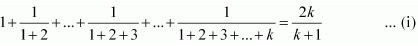We shall now prove that P(k + 1) is true.
Consider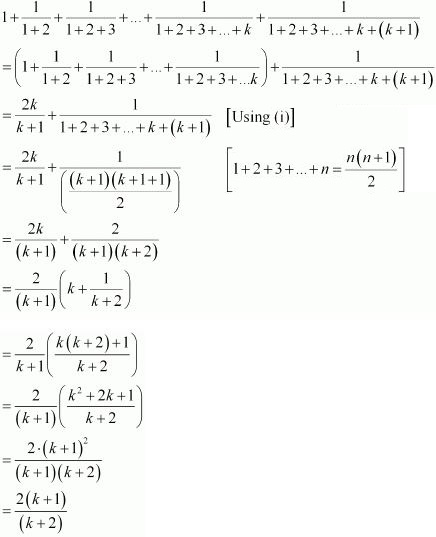Thus, P(k + 1) is true whenever P(k) is true.
Hence, by the principle of mathematical induction, statement P(n) is true for all natural numbers i.e., n.

4. Prove the following by using the principle of mathematical induction for all n ∈ N: 1.2.3 + 2.3.4 + … + n(n + 1) (n + 2) = [n(n + 1)(n + 2)(n +3)]/4
Solution
Let the given statement be P(n), i.e.,
P(n) : 1.2.3 + 2.3.4 + .... + n(n + 1)(n+2) = [n(n+1)(n+2)(n+3)]/4
For n = 1 , we have
P(1) : 1.2.3 = 6 = [1(1 + 1)(1 + 2)(1 + 3)]/4 = (1.2.3.4)/4 = 6, which is true.
Let P(k) be true for some positive integer k, i.e.,
1.2.3 + 2.3.4 + ...+k(k + 1)(k + 2) = [k(k+1)(k + 2)(k + 3)]/4 ...(i)
We shall now prove that P(k + 1) is true.
Consider,
1.2.3 + 2.3.4 + ... +k(k + 1)(k + 2)+ (k+1)(k + 2)(k + 3)
={1.2.3 + 2.3.4 + ....+k(k+1)(k + 2)} + (k + 1)(k + 2)(k + 3)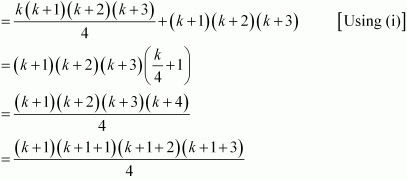Thus, P(k+1) is true whenever P(k) is true .
Hence, by the principle of mathematical induction, statement P(n) is true for all natural numbers i.e., n.

5. Prove the following by using the principle of mathematical induction for all n ∈ N: 1.3 + 2.32 + 3.33 + .... + n.3n =[(2n - 1)3n+1 + 3]/4
Solution
Let the given statement be P(n), i.e.,
P(n) : 1.3 + 2.32 + 3.33 + .... + n.3n  =[(2n - 1)3n+1 + 3]/4
For n = 1, we have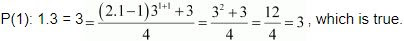Let P(k) be true for some positive integer k, i.e.,
1.3 + 2.32 + 3.33 + .... + k.3k[(2k-1)3k+1 + 3]/4 ...(i)
We shall now prove that P(k + 1) is true.
Consider,
1.3 + 2.32 + 3.33 + .... + k3k = [(2k -1)3k+1 + 3]/4 ...(i)
We shall now prove that P(k + 1)is true.
Consider,
1.3 + 2.32 + 3.33 + ...+k3k + (k +1)3k+1
= (1.3 + 2.32 + 3.33 + .... + k.3k ) + (k + 1)3k+1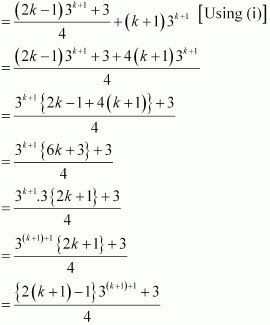Thus, P(k + 1) is true whenever P(k) is true.
Hence, by the principle of mathematical induction, statement P(n) is true for all natural numbers i.e., n.

6. Prove the following by using the principle of mathematical induction for all n ∈ N: 1.2 + 2.3+ 3.4 + ....+ n.(n + 1) = [{n(n+1)(n+2)}/3]
Solution
Let the given statement be P(n), i.e.,
P(n) : 1.2 + 2.3+ 3.4 + .....+ n.(n + 1) = [{n(n+1)(n+2)}/3]
For n = 1, we have
P(1) : 1.2 = 2 = [1(1 +1)(1 + 2)]/3 = 1.2.3/3 = 2 , which is true.
Let P(k) be true for some positive integer k, i.e.,
1.2 + 2.3 + 3.4 + .... + k.(k + 1) = [{k(k+1)(k+2)}/3] ...(i)
We shall now prove that P(k + 1) is true.
Consider
1.2 + 2.3 + 3.4 + ....+ k.(k + 1) + (k + 1).(k + 2)
= [1.2 + 2.3 + 3.4 + ..... + k.(k + 1)] + (k + 1).(k + 2)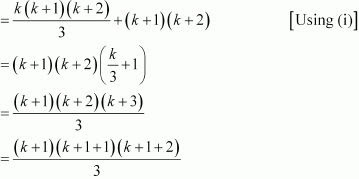Thus, P(k + 1) is true whenever P(k) is true.
Hence, by the principle of mathematical induction, statement P(n) is true for all natural numbers i.e., n.

7. Prove the following by using the principle of mathematical induction for all n ∈ N:  1.3 + 3.5 + 5.7 + .... + (2n - 1)(2n + 1) = [n(4n2 + 6n - 1)]/3

Solution

Let the given statement be P(n) , i.e.,
P(n): 1.3+ 3.5 + 5.7 + ....+(2n - 1)(2n + 1) = [n(4n2 + 6n - 1)]/3
For n = 1, we have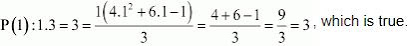Let P(k) be true for some positive integer k, i.e.,
1.3 + 3.5 + 5.7 + ....+(2k - 1)(2k + 1) = [k(4k2 + 6k - 1)]/3 ...(i)
We shall now prove that P(k + 1) is true.
Consider
(1.3 + 3.5 + 5.7 + .... +(2k - 1)(2k + 1) + {2(k + 1) - 1}{2(k+1) +1}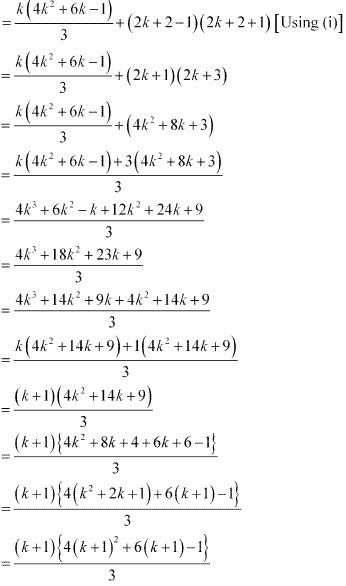Thus, P(k + 1) is true whenever P(k) is true.
Hence, by the principle of mathematical induction, statement P(n) is true for all natural numbers i.e., n.

8. Prove the following by using the principle of mathematical induction for all n ∈ N: 1.2 + 2.22 + 3.22 + … + n.2n = (n – 1) 2n+1 + 2 .

Solution

Let the given statement be P(n), i.e.,
P(n): 1.2 + 2.22 + 3.22 + … + n.2n = (n – 1) 2n+1 + 2
For n = 1, we have
P(1): 1.2 = 2 = (1 – 1) 21+1 + 2 = 0 + 2 = 2, which is true.
Let P(k) be true for some positive integer k, i.e.,
1.2 + 2.22 + 3.22 + … + k.2k = (k – 1) 2k + 1 + 2 …(i)
We shall now prove that P(k + 1) is true.
Consider
{1.2 + 2.22 + 3.23 + ..... +k.2k } + {k + 1}. 2k+1
= (k - 1) 2k+1 + 2 + (k + 1)2k+1
= 2k+1 {(k - 1) + (k + 1)} + 2
= 2k+1  . 2k + 2
= k.2(k+1) + 1  +  2
= {(k + 1) - 1}2(k+1)+1  +  2
Thus, P(k + 1) is true whenever P(k) is true.
Hence, by the principle of mathematical induction, statement P(n) is true for all natural numbers i.e., n.

9. Prove the following by using the principle of mathematical induction for all n ∈ N : 1/2 + 1/4 + 1/8 + .... + 1/2n = 1 - 1/2n

Solution

Let the given statement be P(n), i.e.,
P(n) : 1/2 + 1/4 + 1/8 + .... + 1/2n  = 1 - 1/2n
For n = 1, we have
P(1) : 1/2 = 1 - 1/21  = 1/2 , which is true.
Let P(k) be true for some positive integer k, i.e.,
1/2 + 1/4 + 1/8 + ....+ 1/2k  = 1 - 1/2k  ...(i)
We shall now prove that P(k + 1) is true.
Consider,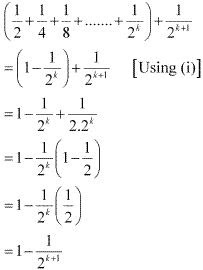Thus, P(k + 1) is true whenever P(k) is true.
Hence, by the principle of mathematical induction, statement P(n) is true for all natural numbers i.e., n.

10. Prove the following by using the principle of mathematical induction for all n ∈ N:  1/2.5 + 1/5.8 + 1/8.11 + .... + 1/[(3n - 1)(3n + 2)] = n/(6n + 4)

Solution

Let the given statement be P(n), i.e.,
P(n) : 1/2.5 + 1/5.8 + 1/8.11 + .... + 1/[(3n - 1)(3n + 2)] = n/(6n + 4)
For n = 1, we have
P(1) = 1/2.5 = 1/10 = 1/(6.1 + 4) = 1/10, which is true.
Let P(k) be true for some positive integer k, i.e.,
1/2.5 + 1/5.8 + 1/8.11 + .... + 1/[(3k - 1)(3k+ 2)] = k/(6k + 4)  ...(i)
We shall now prove that P(k + 1) is true.
Consider,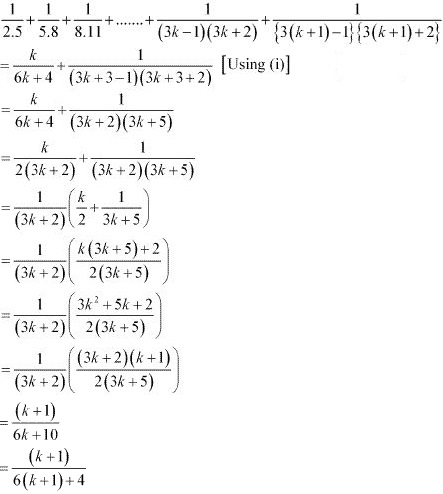Thus, P(k + 1)is true whenever P(k) is true.
Hence, by the principle of mathematical induction, statement P(n) is true for all natural numbers i.e., n.

11. Prove the following by using the principle of mathematical induction for all n ∈ N: 1/(1.2.3) + 1/(2.3.4) + 1/(3.4.5) + ....+ 1/[n(n+1)(n+2)] = n(n+3)/[4(n+1)(n+2)].

Solution

Let the given statement be P(n), i.e,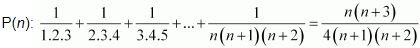For n = 1, we have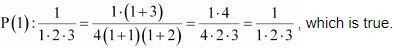Let P(k) be true for some positive integer k, i.e.,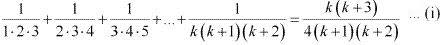We shall now prove that P(k + 1) is true.
Consider,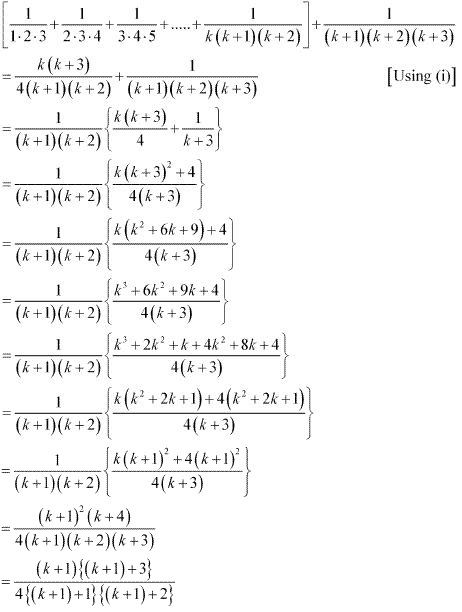Thus, P(k + 1) is true whenever P(k) is true.
Hence, by the principle of mathematical induction, statement P(n) is true for all natural numbers i.e., n.

12. Prove the following by using the principle of mathematical induction for all n ∈ N: a + ar + ar2 + .....+ arn-1 = a(rn - 1)/(r - 1)

Solution

Let the given statement be P(n), i.e.,
P(n): a + ar + ar2 + .....+ arn-1 = a(rn - 1)/(r - 1)
For n = 1, we have
P(1): a =   a(r1 - 1)/(r - 1)  = a , which is true.
Let P(k) be true for some positive integer k, i.e.,
a + ar + ar2 + ....+ ark-1 = a(rk - 1)/(r - 1)  ...(i)
We shall now prove that P(k + 1) is true.
Consider
{a + ar + ar2 + .....+ ark-1 } + ar(k+1)-1
= a(rk - 1)/(r - 1) + ark  [Using (i)]

13. Prove the following by using the principle of mathematical induction for all n ∈ N:
(1+3/1)(1+ 5/4)(1+7/9)...[1 + (2n+1)/n2 ) = (n+ 1)2

Solution

Let the given statement be P(n), i.e.,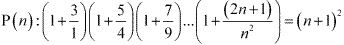For n = 1, we have
P(1): (1 + 3/1) = 4 = (1 + 1)2 = 22 = 4, which is true.
Let P(k) be true for some positive integer k, i.e.,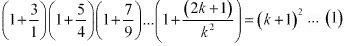We shall now prove that p(k + 1) is true.
Consider,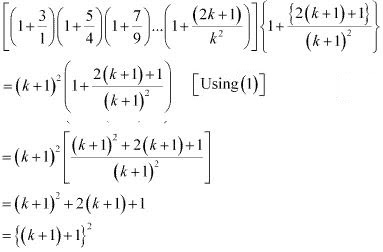Thus, P(k + 1) is true whenever P(k) is true.
Hence, by the principle of mathematical induction, statement P(n) is true for all natural numbers i.e., n.

14. Prove the following by using the principle of mathematical induction for all n ∈ N
(1 + 1/1)(1 + 1/2)(1+ 1/3).....(1 + 1/n) = (n + 1)

Solution

Let the given statement be P(n), i.e.,
P(n) : (1 + 1/1)(1 + 1/2)(1+ 1/3).....(1 + 1/n) = (n + 1)
For n = 1, we have
P(1): (1 + 1/1) = 2 = (1 + 1) , which is true.
Let P(k) be true for some positive integer k, i.e.,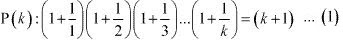We shall now prove that P(k + 1) is true.
Consider,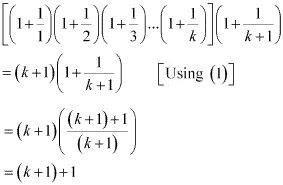Thus, P(k + 1) is true whenever P(k) is true.
Hence, by the principle of mathematical induction, statement P(n) is true for all natural numbers i.e., n.

15. Prove the following by using the principle of mathematical induction for all n ∈ N
12 + 32 + 52 + ... + (2n - 1)2 = [n(2n - 1)(2n+1)]/3

Solution

Let the given statement be P(n), i.e.,
P(n) = 12 + 32 + 52 + ... + (2n - 1)2 = [n(2n - 1)(2n+1)]/3
For n = 1, we have
P(1) = 12 = 1 = [1(2.1 - 1)(2.1 + 1)]/3 = 1.1.3/3 = 1, which is true.
Let P(k) be true for some positive integer k, i.e.,
P(k) = 12 + 32 + 52 + ...+(2k - 1)2  = [k(2k-1)(2k+1)]/3 ...(1)
We shall now prove that P(k+1) is true.
Consider,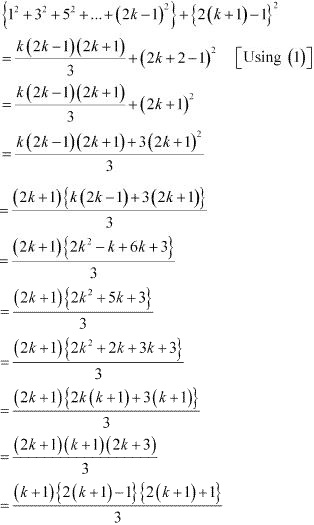Thus, P(k + 1) is true whenever P(k) is true.
Hence, by the principle of mathematical induction, statement P(n) is true for all natural numbers i.e., n.

16. Prove the following by using the principle of mathematical induction for all n ∈ N
1/1.4 + 1/4.7 + 1/7.10 + ... + 1/[(3n-2)(3n+1)] = n/(3n+1)

Solution

Let the given statement be P(n), i.e.,
P(n) : 1/1.4 + 1/4.7 + 1/7.10 + ... + 1/[(3n-2)(3n+1)] = n/(3n+1)
For n = 1, we have
P(1) = 1/1.4 = 1/(3.1 + 1) = 1/4 = 1/1.4 , which is true.
Let P(k) be true for some positive integer k, i.e.,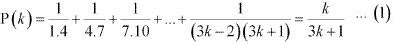We shall now prove that P(k+1) is true.
Consider,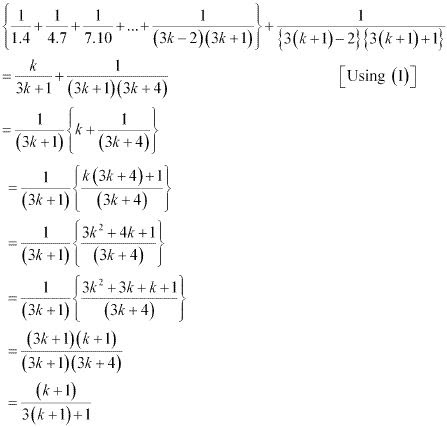Thus, P(k + 1) is true whenever P(k) is true.
Hence, by the principle of mathematical induction, statement P(n) is true for all natural numbers i.e., n.

17. Prove the following by using the principle of mathematical induction for all n ∈ N
1/3.5 + 1/5.7 + 1/7.9 + ....+ 1/[(2n+1)(2n+3)] = n/3(2n+3)

Solution

Let the given statement be P(n), i.e.,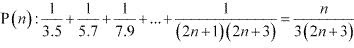For n = 1, we have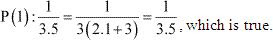Let P(k) be true for some positive integer k, i.e.,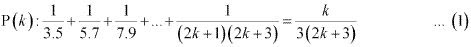We shall now prove that P(k + 1) is true.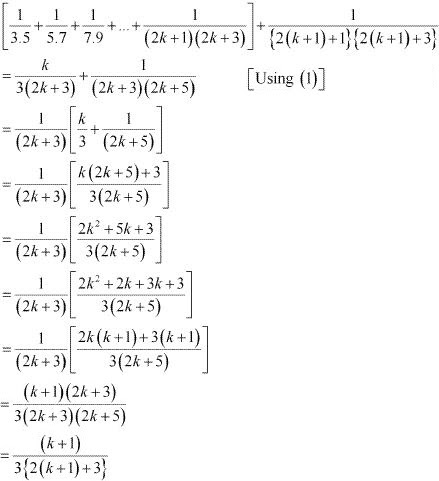Thus, P(k + 1) is true whenever P(k) is true.
Hence, by the principle of mathematical induction, statement P(n) is true for all natural numbers i.e., n.

18. Prove the following by using the principle of mathematical induction for all n ∈ N: 1+ 2 + 3 + ... + n <(1/8)(2n + 1)2.

Solution

Let P(k) be true for some positive integer k, i.e.,
1+2+....+ k < (1/8)(2n + 1)2
Adding (k + 1) on both the sides of the inequality , we have,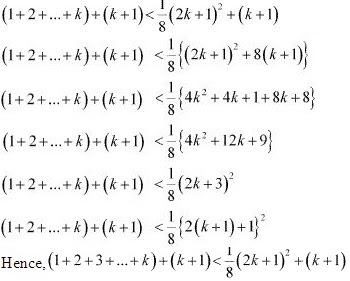Thus, P(k + 1) is true whenever P(k) is true.
Hence, by the principle of mathematical induction, statement P(n) is true for all natural numbers i.e., n.

19. Prove the following by using the principle of mathematical induction for all n ∈ Nn (n + 1) (n + 5) is a multiple of 3.

Solution

Let the given statement be P(n), i.e.,
P(n): n (n + 1) (n + 5), which is a multiple of 3.
It can be noted that P(n) is true for n = 1 since 1 (1 + 1) (1 + 5) = 12, which is a multiple of 3.
Let P(k) be true for some positive integer k, i.e.,
k (k + 1) (k + 5) is a multiple of 3.
∴ k (k + 1) (k + 5) = 3m, where m ∈ N …(1)
We shall now prove that P(k + 1) is true whenever P(k) is true.
Consider
(k + 1){(k+1) + 1}{(k+1) + 5}
= (k+1)(k+2){(k+5) + 1}
= (k + 1)(k + 2)(k+5)+(k+1)(k+2)
= {k(k+1)(k+5)+2(k+1)(k+5)}+ (k+1)(k+2)
= 3m + (k + 1){2(k+5)+(k+2)}
= 3m + (k+1){2k+10+k+2}
= 3m+ (k+1)(3k+12)
= 3m+ 3(k+1)(k+4)
= 3[m + (k+1)(k+4)] = 3 × q, where q = {m+(k+ 1)(k+4)} is some natural number
Therefore, (k+1)[(k+1)+1][(k+1) + 5] is a multiple of  3.
thus, P(k + 1) is true whenever P(k) is true.
Hence, by the principle of mathematical induction, statement P(n) is true for all natural numbers i.e., n.

20. Prove the following by using the principle of mathematical induction for all n ∈ N: 102n–1 + 1 is divisible by 11.

Solution

Let the given statement be P(n), i.e.,
P(n): 102n–1 + 1 is divisible by 11.
It can be observed that P(n) is true for n = 1 since P(1) = 102.1–1 + 1 = 11, which is divisible by 11.
Let P(k) be true for some positive integer k, i.e.,
102k–1 + 1 is divisible by 11.
∴102k–1 + 1 = 11m, where m ∈ N …(1)
We shall now prove that P(k + 1) is true whenever P(k) is true.
Consider
102(k+1)-1 + 1
= 102k+2-1 + 1
= 102k+1 + 1
= 102 (102k-1 + 1 - 1) + 1
= 102 (102k-1 + 1) - 102 + 1
= 102 .11 m - 100 + 1 [Using (1)]
= 100 × 11m - 99
= 11(100m - 9)
= 11r, where r = (100m - 9) is some natural number
Therefore, 102(k+1)-1 + 1 is divisible by 11.
Thus, p(k + 1) is true whenever P(k) is true.
Hence, by the principle of mathematical induction, statement P(n) is true for all natural numbers i.e., n.

21. Prove the following by using the principle of mathematical induction for all n ∈ Nx2n – y2n is divisible by x y.

Solution

Let the given statement be P(n), i.e.,
P(n): x2n – y2n is divisible by x + y.
It can be observed that P(n) is true for n = 1.
This is so because x× 1 – y× 1 = x2 – y2 = (x + y) (x – y) is divisible by (x + y).
Let P(k) be true for some positive integer k, i.e.,
x2k – y2k is divisible by x + y.
∴x2k – y2k = m (x + y), where m ∈ N …(1)
We shall now prove that P(k + 1) is true whenever P(k) is true.
Consider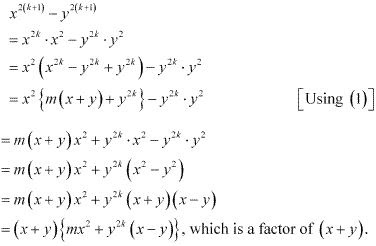Thus, P(k + 1) is true whenever P(k) is true.
Hence, by the principle of mathematical induction, statement P(n) is true for all natural numbers i.e., n.

22. Prove the following by using the principle of mathematical induction for all n ∈ N: 32n+2 – 8n– 9 is divisible by 8.

Solution

Let the given statement be P(n), i.e.,
P(n): 32n+2 – 8n – 9 is divisible by 8.
It can be observed that P(n) is true for n = 1 since 3× 1 + 2 – 8 × 1 – 9 = 64, which is divisible by 8.
Let P(k) be true for some positive integer k, i.e.,
32k+2 – 8k – 9 is divisible by 8.
∴32k+2 – 8k – 9 = 8m; where m ∈ N …(1)
We shall now prove that P(k + 1) is true whenever P(k) is true.
Consider
32(k+1)+2 - 8(k+1) - 9
= 32k+2.32  -8k-8-9
= 32 (32k+2  -8k - 9 + 8k + 9) - 8k - 17
= 32 (32k+2 - 8k - 9) + 32 (8k + 9) - 8k - 17
= 9.8m + 9(8k + 9) - 8k - 17
= 9.8m + 72k + 81 - 8k - 17
= 9.8m + 64k + 64
= 8(9m + 8k + 8)
= 8r, where r = (9m + 8k + 8) is a natural number
Therefore, 32(k+1)+2 - 8(k+1) - 9 is divisible by 8.
Thus, P(k + 1) is true whenever P(k) is true.
Hence, by the principle  mathematical induction, statement P(n) is true for all natural number  i.e., n.

23. Prove the following by using the principle of mathematical induction for all n ∈ N: 41n – 14n is a multiple of 27.

Solution

Let the given statement be P(n), i.e.,
P(n):41n – 14nis a multiple of 27.
It can be observed that P(n) is true for n = 1 since 411 - 141 = 27, which is a multiple of 27.
Let P(k) be true for some positive integer k, i.e.,
41k – 14kis a multiple of 27
∴41k – 14k = 27m, where m ∈ N …(1)
We shall now prove that P(k + 1) is true whenever P(k) is true.
Consider
41k+1 - 14k+1
= 41k . 41- 14k .14
= 41(41k - 14k + 14k ) - 14k .14
= 41(41k - 14k ) + 41.14k - 14k .14
= 41.27m + 14k (41-14)
= 41.27m + 27.14k
= 27(41m - 14k )
= 27× r, where r = (41m - 14k ) is a natural number
Therefore, 41k+1 - 14k+1 is a multiple of 27.
Thus, P(k+1) is true whenever P(k) is true.
Hence, by the principle of mathematical induction, statement P(n) is true for all natural numbers i.e., n.

24. Prove the following by using the principle of mathematical induction for all n ∈ N (2+7) < (n + 3)2

Solution

Let the given statement be P(n), i.e.,
P(n): (2+7) < (n + 3)2
It can be observed that P(n) is true for n = 1 since 2.1 + 7 = 9 < (1 + 3)2 = 16, which is true.
Let P(k) be true for some positive integer k, i.e.,
(2k + 7) < (k + 3)2 …(1)
We shall now prove that P(k + 1) is true whenever P(k) is true.
Consider
[2(k+1)+7] = (2k + 7) + 2
∴ [2(k+1)+7] = (2k+7)+2 <(k+3)2 + 2  [using (1)]
⇒ 2(k+1) + 7 < k2 + 6k + 9 + 2
⇒ 2(k+1) + 7 < k2 + 6k + 11
Now, k2 + 6k + 11 < k2 + 8k + 16
∴ 2(k+1) + 7 < (k + 4)2
2(k + 1) + 7 < {(k+1) + 3}2
Thus, P(k + 1) is true whenever P(k) is true.
Hence, by the principle of mathematical induction, statement P(n) is true for all natural number i.e., n.